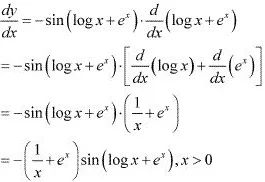# Class 12 Maths NCERT Solutions for Chapter 5 Continuity and Differentiability Exercise 5.4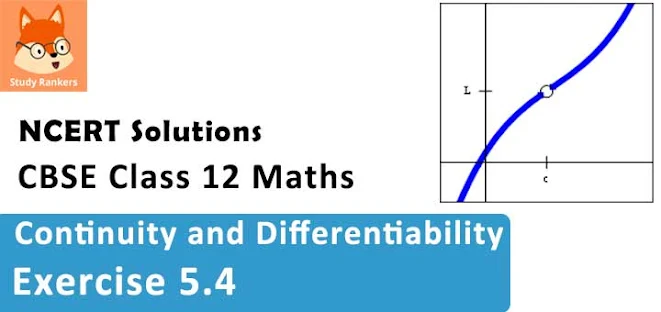### Continuity and Differentiability Exercise 5.4 Solutions

1. Differentiate the following w.r.t. x :
ex /sin x

Solution

Let y = ex /sin x
By using the quotient rule, we obtain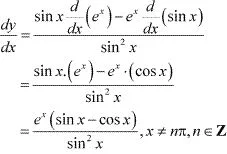2. Differentiate the following w.r.t. x : esin-1 x

Solution
Let y = esin-1 x
By using the chain rule, we obtain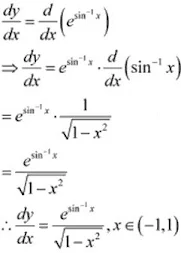3. Differentiate the following w.r.t. x: ex

Solution

Let y = ex
By using the chain rule, we obtain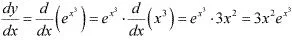4. Differentiate the following w.r.t. x :
sin(tan-1 e-x )

Solution

Let y = sin(tan-1 e-x )
By using the chain rule, we obtain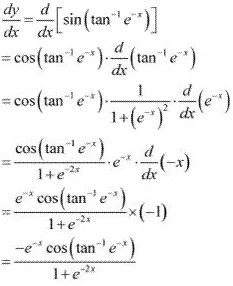5. Differentiate the following w.r.t. x :
log(cos ex )

Solution

Let y = log(cos ex )
By using the chain rule, we obtain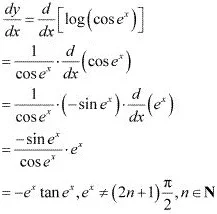6. Differentiate the following w.r.t. x :

ex + ex2 + ex3

Solution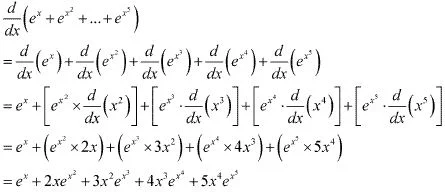7. Differentiate the following w.r.t. x :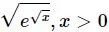Solution
Let y =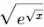y2 = e√x
By differentiating this relationship with respect to x, we obtain
y2 = e√x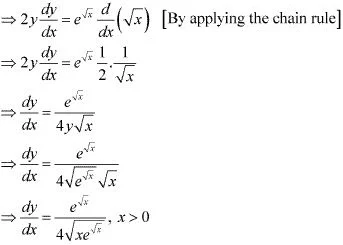8. Differentiate the following w.r.t. x : log (log x), x > 1
Solution
Let y = log (log x)
By using the chain rule, we obtain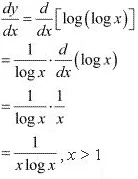9. Differentiate the following w.r.t. x :
cos x/log x , x > 0
Solution
Let y = cos x/log x
By using the quotient rule, we obtain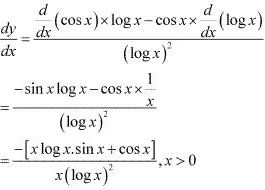10. Differentiate the following w.r.t. x :
cos (log x + ex ), x > 0
Solution
Let y = cos (log x + ex )
By using the chain rule, we obtain